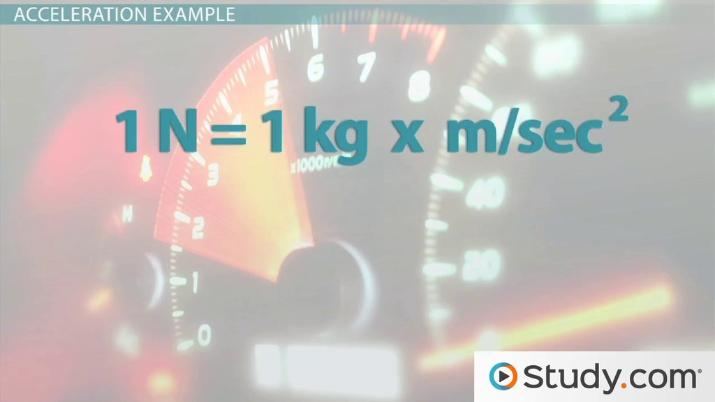Newton's Second Law of Motion: The Relationship Between Force and Acceleration

Lesson Transcript
Instructor: John Simmons

John has taught college science courses face-to-face and online since 1994 and has a doctorate in physiology.

Newton's second law of motion is related to acceleration and force. Learn about net force, implications for this particular law of motion, and calculations for acceleration and force for moving objects. Updated: 08/24/2021

Newton's Second Law of Motion

Consider two balls, one with a mass of 1 kg and the other with a mass of 10 kg. Which ball would experience a greater change in motion if kicked with the same force? Clearly, the smaller ball would experience a greater change in motion. An object's state of motion can be described as its velocity, where velocity is the speed of an object with respect to its direction. Objects at rest - for example, the balls you see on the screen - have zero velocity. Once kicked, the ball's state of motion changes. In other words, its velocity changes. When an object changes its velocity, it has what we call acceleration. Newton's second law of motion provides the explanation for the behavior of objects when forces are applied. The law states that external forces cause objects to accelerate, and the amount of acceleration is directly proportional to the net force acting on the objects and inversely proportional to the mass of the objects.An error occurred trying to load this video.

Try refreshing the page, or contact customer support.

Coming up next: Newton's Third Law of Motion: Examples of the Relationship Between Two Forces

You're on a roll. Keep up the good work!

Replay
Your next lesson will play in 10 seconds
• 0:08 Newton's Second Law of Motion
• 1:15 What is Net Force?
• 2:28 How to Calculate for…
• 4:26 How to Calculate for Force
• 5:34 Implications of…
• 6:40 Lesson Summary
Save Save

Want to watch this again later?

Timeline
Autoplay
Autoplay
Speed Speed

What is Net Force?

Notice that Newton's second law states that the amount of acceleration is directly proportional to the net force acting on the object. What is the net force and how do we calculate it? Net force is the sum of all forces acting on an object in a particular direction. Since forces have direction, they are vector quantities. Vector quantities are fully described with both magnitude and direction and are represented with arrows. Consider an object being pushed to the left with 10 Newtons of force and to the right with 5 Newtons of force. The net force = 5 N to the left as 10 N - 5 N = 5 N. The forces are subtracted from each other since they are pointing in opposite directions. The forces would be added to each other if they were pointing in the same direction. Once the net force is determined, the acceleration of the object can be determined.

How to Calculate for Acceleration

Acceleration is a change in velocity. As long as we know the mass of the object and the net force acting on the object, we can determine acceleration. Let's look at the formula:

a = f (net) / m, where a = acceleration, f (net) = the net force acting on the object, m = the mass of the object.

Acceleration Example

Let's look at an example. Consider a 10 kg object forced to the left with 10 Newtons and to the right with 20 Newtosn. What is the acceleration of this object?

Let's recall the formula for acceleration. a = f (net) / m

Let's first calculate the net force. As the forces are acting in opposite directions, we subtract them.

f (net) = 20 N right - 10 N left, and we getf (net) = 10 N right

Now, plug net force into the equation with mass to calculate for acceleration.

a = 10 N / 10 kg or a = 1 N/Kg

A Newton of force equals 1 kg * m/sec^2. 1 N = 1 kg * m/sec^2. If we substitute this value of a Newton into the equation, mass (kg) is cancelled out, and that leaves us with the units m/sec^2. Those are the units for acceleration.

Therefore the acceleration of our object is, a = 1 m/sec^2.

How to Calculate for Force

If we know the mass and acceleration of an object, we can calculate for force. Simply rearrange the equation to solve for force. So, as you see on the screen, f (net) = m * a.

Force Example

Consider again our 10 kg object, now moving to the west with an acceleration of 10 m/sec^2. What is the net force acting on this object?

To unlock this lesson you must be a Study.com Member.

Register to view this lesson

Are you a student or a teacher?